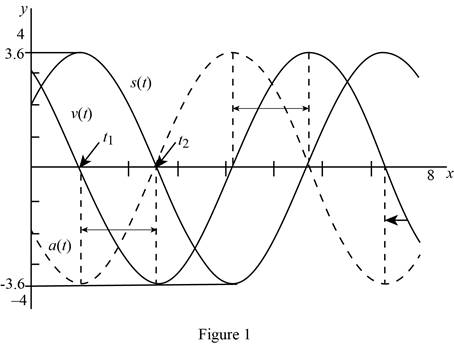# The velocity and acceleration at time t .### Single Variable Calculus: Concepts...

4th Edition
James Stewart
Publisher: Cengage Learning
ISBN: 9781337687805### Single Variable Calculus: Concepts...

4th Edition
James Stewart
Publisher: Cengage Learning
ISBN: 9781337687805

#### Solutions

Chapter 3.3, Problem 36E

(a)

To determine

## To find: The velocity and acceleration at time t.

Expert Solution

The velocity of motion at time t is 2sint+3cost_.

The acceleration of motion at time t is 2cost3sint_.

### Explanation of Solution

Given:

The equation of motion is s(t)=2cost+3sint, where t is in seconds and x is in centimeters.

Derivative rule:

(1) Sum Rule: ddt[f(t)+g(t)]=ddt[f(t)]+ddt[g(t)]

(2) Constant Multiple Rule: ddt[cf(t)]=cddt[f(t)]

Recall:

If x(t) is a displacement of a particle and the time t is in seconds, then the velocity of the particle is v(t)=dsdt.

If v(t) is a velocity of the particle and the time t is in seconds, then the acceleration of the particle is a(t)=dvdt.

Calculation:

Obtain the velocity at time t.

v(t)=ddt(s(t))=ddt(2cost+3sint)

Apply the sum rule (1),

v(t)=ddt[2cost]+ddt[3sint]

Apply the constant multiple rule (2),

v(t)=2ddt[cost]+3ddt[sint]=2[sint]+3[cost]=2sint+3cost

Thus, the velocity of  x(t)=2cost+3sint at time t is v(t)=2sint+3cost_.

Obtain the acceleration at time t.

a(t)=ddt(v(t))=ddt(2sint+3cost)

Apply the sum rule (1)

a(t)=ddt[2sint]+ddt[3cost]

Apply the constant multiple rule (2)

a(t)=2ddt[sint]+3ddt[cost]=2[cost]+3[sint]=2cost3sint

Therefore, the acceleration of  x(t)=2cost+3sint at time t is a(t)=2cost3sint_.

(b)

To determine

Expert Solution

### Explanation of Solution

From part (a), the velocity and acceleration functions are v(t)=2sint+3cost and a(t)=2cost3sint, respectively.

Use online graphing calculator and draw graph the velocity and acceleration functions as shown below in Figure 1.From Figure1, it is observed that the maximum position of the mass travel is approximately 3.6 cm and the minimum position of the mass travel is approximately 3.6 cm.

(c)

To determine

### To find: When the mass passes through the equilibrium position for the first time.

Expert Solution

The mass passes through the equilibrium position for the first time is t2.55 s.

### Explanation of Solution

Definition used:

The mass is in equilibrium when its acceleration is zero.

Calculation:

The equation of motion is s(t)=2cost+3sint.

From (a), the acceleration of  x(t) at time t is a(t)=2cost3sint.

Use the definition stated above and solve for a(t)=0.

2cost3sint=03sint=2costsintcost=23tant=23

Multiply the equation by tan1,

tan1(tant)=tan1(23)t=tan1(23)0.5880

Therefore, the mass of equilibrium at t=0.5880+nπ, where n is an integer.

Compute t when n=1.

t=0.5880+π2.5536

Hence, the mass passes through the equilibrium for the first time is t2.55 s.

(d)

To determine

### To find: The distance from the equilibrium position to the final position.

Expert Solution

The mass travels about 3.6056 cm from its equilibrium position.

### Explanation of Solution

From Figure1, the maximum position is t1 s when v=0.

From part (a), v(t)=2sint+3cost.

Set v(t)=0 and solve for t.

2sint+3cost=02sint=3costsintcost=32tant=32

Multiply the equation by tan1,

tan1(tant)=tan1(32)t=tan1(32)t0.9828

Obtain v(t) when t0.9828.

s(0.9828)=2cos(0.9828)+3sin(0.9828)3.6056

Thus, the mass travels about 3.6056 cm from its equilibrium position.

(e)

To determine

### To find: When is the speed greatest?

Expert Solution

The speed is maximum at t=2.55+nπ.

### Explanation of Solution

Note that, the velocity is zero when the mass reaches its maximum or minimum and the speed will be greatest when the mass passess through the equilibrium position.

That is, s(t)=0.

2cost+3sint=03sint=2costsintcost=23tant=23

Multiply the equation by tan1,

tan1(tant)=tan1(23)t=tan1(23)

For each additional rotation of π, the speed will be greatest when t=tan1(23)+nπ, n is an integer.

### Have a homework question?

Subscribe to bartleby learn! Ask subject matter experts 30 homework questions each month. Plus, you’ll have access to millions of step-by-step textbook answers!